# USB-Based Temperature Monitor

By Analog Devices, Inc.

Contributed By Convergence Promotions LLC

Using an analog MCU, and LDO, an external thermistor, and a few discretes, you can construct a highly accurate temperature sensing application.

The circuit in Figure 1 shows how the Analog Devices ADuC7122 precision analog microcontroller can be used in an accurate thermistor temperature monitoring application. The ADuC7122 integrates a multichannel 12-bit SAR ADC, twelve 12-bit DACs, a 1.2 V internal reference, as well as an ARM7 core, 126 kB flash, 8 kB SRAM, and various digital peripherals, such as UART, timers, SPI, and two I2C interfaces. The ADuC7122 is connected to a 4.7 kΩ thermistor.Figure 1: ADuC7122 used as a temperature monitor interfaced to a thermistor (simplified schematic, all connections not shown).

Due to the small form factor of the ADuC7122 (7 mm × 7 mm, 108-ball BGA package) the entire circuit will fit on an extremely small PCB, thus further reducing cost.

Similar in function to an RTD, thermistors are low-cost, temperature-sensitive resistors and are constructed of solid semiconductor materials, which exhibit a positive or negative temperature coefficient. Thermistors are inexpensive and have high sensitivity. They detect small variations in temperature, which could not be observed with an RTD or a thermocouple. However, thermistors are highly nonlinear; thus, they are limited to applications with very narrow temperature ranges if linearization techniques are not applied. Circuit linearization techniques can be accomplished in software.

Despite the powerful ARM7 core and high-speed SAR ADCs, the ADuC7122 still provides a low-power solution. With the ARM7 core running at 326.4 kHz and the primary ADC active and measuring the external temperature sensor, the entire circuit typically consumes 7 mA. Between temperature measurements, the ADC and/or the microcontroller can be switched off to further minimize power consumption.

Circuit description

The circuit shown in Figure 1 is powered entirely from the USB interface. The 5 V supply from the USB is regulated to 3.3 V using the ADP3333 (3.3V) low-dropout linear regulator. The regulated 3.3 V supplies the DVDD voltage to the ADuC7122. The AVDD supply to the ADuC7122 has additional filtering as shown. A filter is also placed on the USB supply at the input of the linear regulator.

The following features of the ADuC7122 are used in this application:

• ARM7TDMI core: The powerful 16-/32-bit ARM7 core with integrated 126 kB flash and SRAM memory, runs the user code that configures and controls the ADC, processes the ADC conversions from the thermistor sensor, and controls the communications over the UART/USB interface.
• UART: The UART is used as the communication interface to the host PC.
• Two external switches/buttons (not shown) are used to force the part into its flash boot mode. By holding DOWNLOAD low and toggling the RESET switch, the ADuC7122 will enter boot mode instead of normal user mode. In boot mode, the internal flash may be reprogrammed through the I2CWSD tool utilizing the USB interface.
• BUF_VREF: The band gap reference also connects through buffers to the BUF_VREF1 and the BUF_VREF2 pins, which can be used as a reference for other circuits in the system. A minimum of 0.1 µF capacitor should be connected to these pins to reduce noise.

The thermistor used in the circuit is a 4.7 kΩ resistor, model number NCP18XM472. It is available in a 0603 surface-mount package. The thermistor used in the circuit in Figure 2 has the following specifications at 25°C: ß = 3500 (the ß parameter describes resistance as a function of temperature) and resistance (R25) = 4.7 kΩ.Figure 2: A simple temperature sensor circuit implemented with the ADuC7122.

The USB interface to the ADuC7122 is implemented with an FT232R UART to USB transceiver, which converts USB signals directly to the UART protocol.

In addition to the decoupling shown in Figure 1, the USB cable itself should have a ferrite for added EMI/RFI protection. The ferrite beads used in the circuit are Taiyo Yuden, BK2125HS102-T, which have an impedance of 1,000 Ω at 100 MHz.

The circuit must be constructed on a multilayer PC board with a large area ground plane. Proper layout, grounding, and decoupling techniques must be used to achieve optimum performance.

The input thermistor circuit in Figure 2 is designed to produce accurate temperature measurements from 0°C to 90°C. Note that this system contains no temperature calibration. This circuit contains a simple thermistor circuit that does not contain circuit linearization. If this circuit employed linearization techniques, it could function over a broader range of temperatures; however, this would decrease the resolution of the sensor.

The circuit in Figure 2 is set up in a voltage divider configuration. This will allow us to transform the ADC result, D, into a measurement of the resistance of RTH (thermistor) using the following formulas: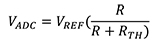Equation 1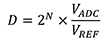Equation 2

Substitute Equation 1 in to Equation 2 and simplify: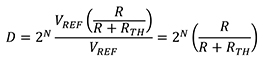Equation 3

Solve Equation 3 for RTH and rearrange: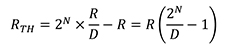Equation 4

Once the resistance of the thermistor is calculated, the Steinhart-Hart equation can be used to determine the current temperature of the sensor. The traditional form of the Simplified ß Parameter variation of the Steinhart-Hart equation would be: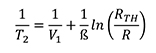Equation 5

Solve Equation 5 for T2 and rearrange: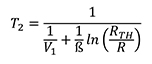Equation 6

Substituting Equation 4 into Equation 6 and simplifying, the ADuC7122 is able to determine the sensor temperature using the following formula: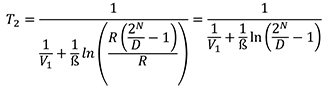Equation 7

Where:

 T2 =unknown temperature V1 =298K β =β parameter of the thermistor @ 298K or 25°C. β=3500 R25 =resistance of thermistor @ 298K or 25°C. R25=4.7kΩ RTH =resistance of thermistor @ unknown temperature as calculated by formula above

Figure 3 plots the response of the ADuC7122 to the thermistor sensor detailed in Figure 2 over temperature.Figure 3: ADuC7122 thermistor sensor measured output (converted to volts) with ADCO versus temperature.

Code description

The source code and a HyperTerminal configuration file used to test the attached circuit can be downloaded as a zip file at www.analog.com/CN0153_Source_Code.

The UART is configured for a baud rate of 9600, 8 data bits, no parity and no flow control. If the circuit is connected directly to a PC, a communication port viewing application such as HyperTerminal can be used to view the results sent by the program to the UART (Figure 4). The source code is commented to make it easier to understand and manipulate. The code was compiled and tested using the Keil µVision 3 application.Figure 4: Output of HyperTerminal communication port viewing application.

Common variations

The ADP3333 (3.3 V) can be replaced with the ADP120 (2.5 V), which has a wider operating temperature range (-40°C to +125°C) and consumes less power (typically 20 µA versus 70 µA) but has a lower maximum input voltage range (5.5 V versus 12 V). Note that the ADuC7122 can be programmed or debugged using a standard JTAG interface. For a standard UART to RS-232 interface, the FT232R transceiver can be replaced with a device such as the ADM3202, which requires a 3 V power supply.

The thermistor circuit described here can be adapted to operate with other precision analog microcontrollers, such as the ADuC7020 series, the ADuC7023, and the ADuC7061 series.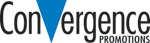Disclaimer: The opinions, beliefs, and viewpoints expressed by the various authors and/or forum participants on this website do not necessarily reflect the opinions, beliefs, and viewpoints of Digi-Key Electronics or official policies of Digi-Key Electronics.